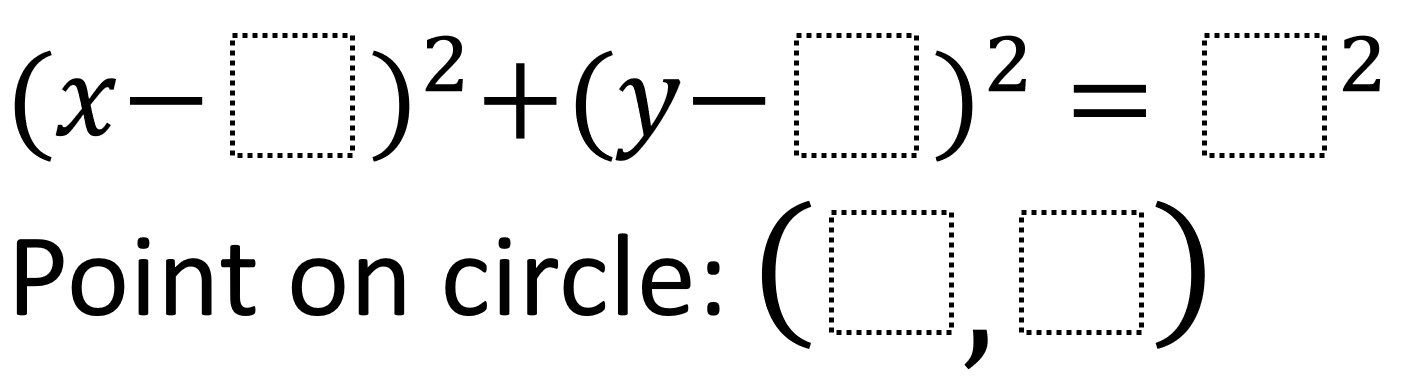# Equations of Circles 1

Directions: Using the integers -9 to 9, at most one time each, fill in the boxes to create a circle and a point on the circle.### Hint

How can you use the values being subtracted from x and y to adjust where the circle is?

For an interactive conceptual version of this problem created in GeoGebra, click here

There are many potential answers but all will come from a circle with a radius of 5 units including:
(x – -2)^2 + (y – -1)^2 = 5^2
Point on circle: (-6, -4)

(x – -2)^2 + (y – -1)^2 = 5^2
Point on circle: (1, 3)

Source: Robert Kaplinsky

## Similar Triangles 2

Directions: Using the digits 0 to 9 at most one time each, create two similar …